# Ford-Fulkerson 最大流求解方法

• 怎样搜索到一条增广路？
• 如何利用搜索到的增广路求解最大流问题？
• Ford-Fulkerson 算法求最大流的原理是什么？

# 如何求得最大流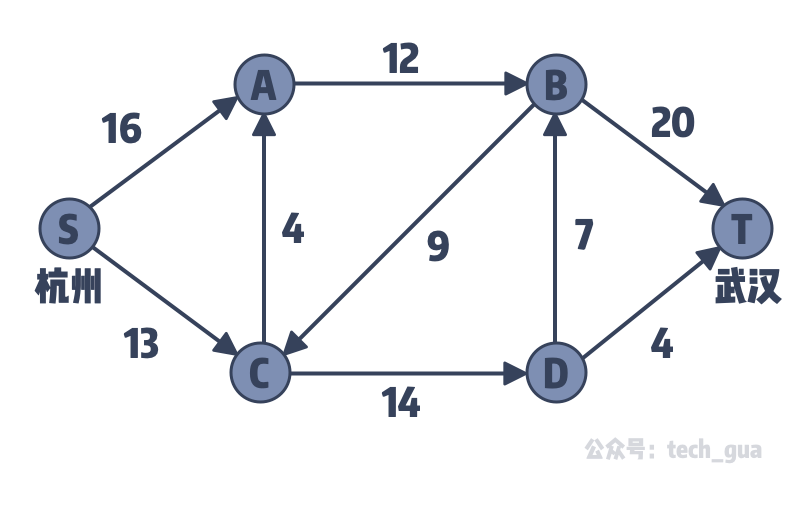1. 搜索出一条增广路；
2. 在这条路径中所有的边容量减去这条增广路的流量，如果容量为 0 则拆边；
3. 返回操作一，如果没有增广路则得到答案。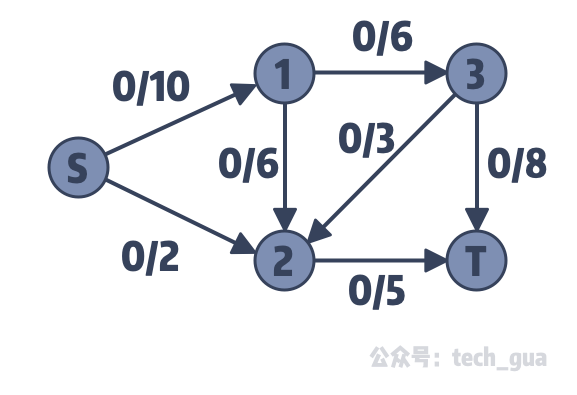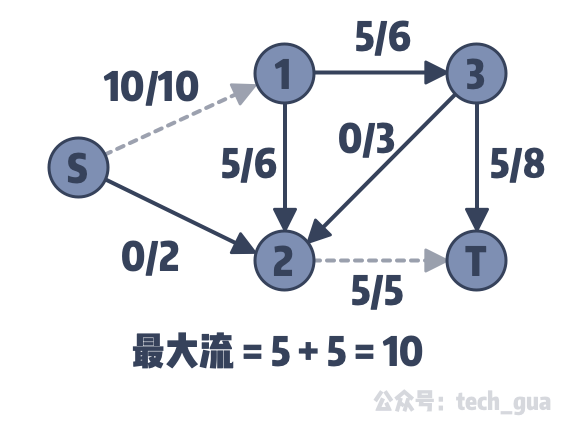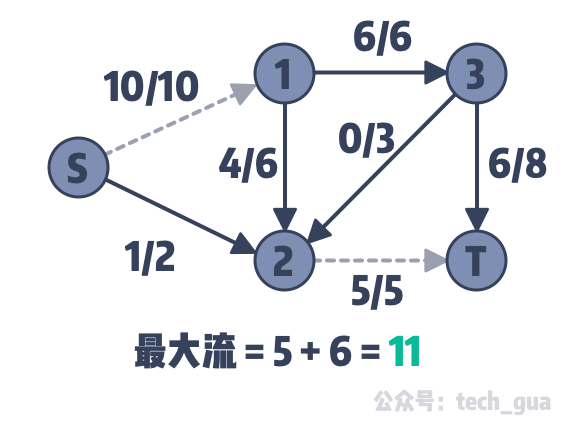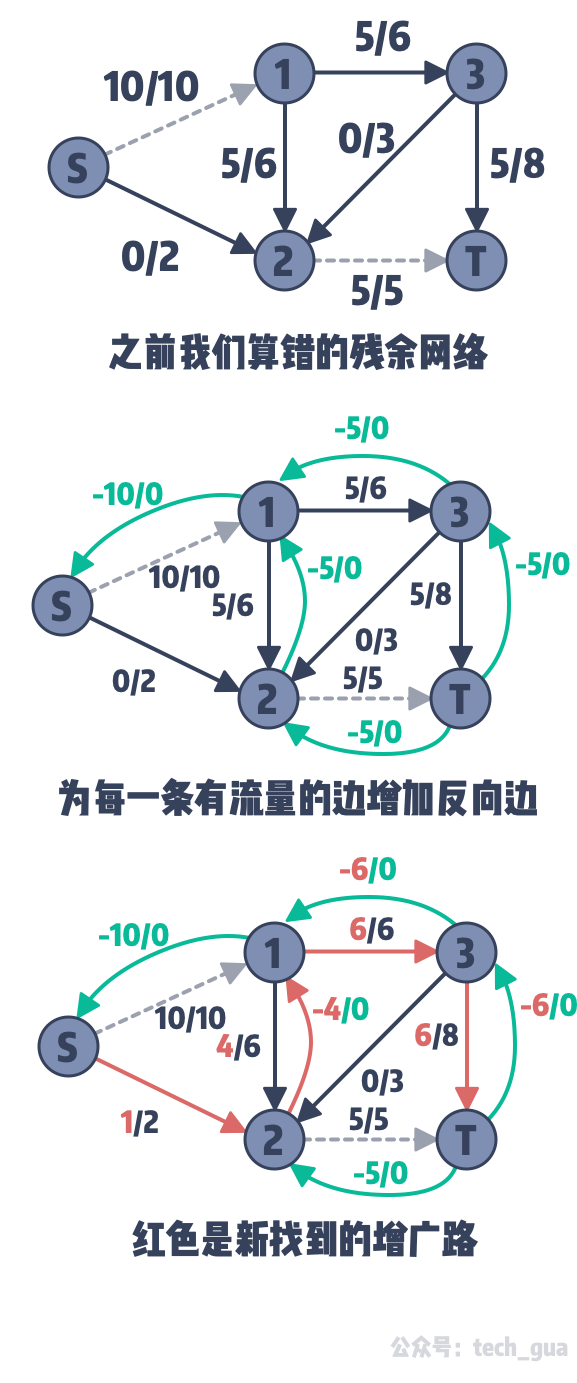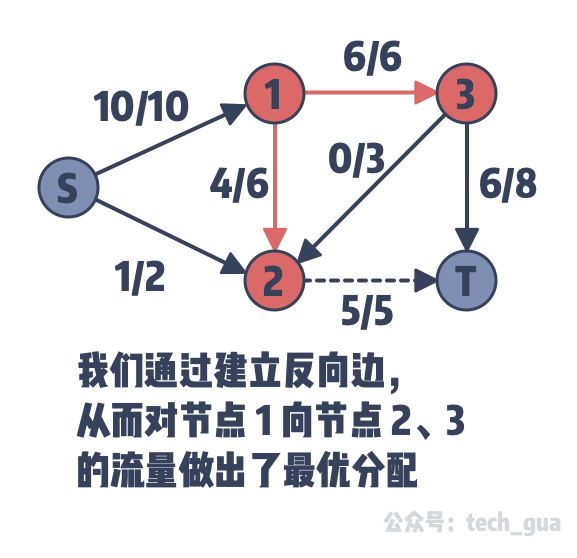1. 搜索出一条增广路；
2. 在这条路径中所有的边容量减去这条增广路的流量，并建立流量为增广路增加流量相反数的反向边
3. 返回操作一，如果没有增广路则得到答案。

# 如何代码实现查找增广路？

/**
* 查找增广路
* @param c 当前节点
* @param t 汇点
* @param f 当前路径中的容量最小值
* @return
*/
int dfs(int c, int t, int f) {
// 如果当前节点是汇点 t，直接返回容量最小值，即增广路增加的流量
if (c == t) {
return f;
}
// 记忆化搜索染色
used[c] = true;
// 遍历 c 节点下一个节点
for (int i = 0; i < G[c].size(); ++ i) {
Edge &e = G[c][i];
// 如果这个节点未被访问到，并且其当前容量大于 0
if (!used[e.to] && e.cap > 0) {
// 访问到最深层节点
int d = dfs(e.to, t, min(f, e.cap));
if (d > 0) {
// 当前边容器减少
e.cap -= d;
// 反向边容量增加
G[e.to][e.rev].cap += d;
return d;
}
}
}
return 0;
}


# 一些边沿知识# Ford-Fulkerson 方法的 代码实现

#include <iostream>
#include <string>
#include <vector>
#include <regex>
using namespace std;

const int MAX_V = 1e4 + 5;

struct Edge {
// 终点、容量、反向边
int to, cap, rev;
Edge(int _to, int _cap, int _rev): to(_to), cap(_cap), rev(_rev) {}
};

vector<Edge> G[MAX_V];
bool used[MAX_V];

void add_edge(int from, int to, int cap) {
G[from].push_back(Edge(to, cap, G[to].size()));
G[to].push_back(Edge(from, 0, G[from].size() - 1));
}

/**
*
* @param c 当前节点
* @param t 汇点
* @param f 当前路径中的容量最小值
* @return
*/
int dfs(int c, int t, int f) {
if (c == t) {
return f;
}
used[c] = true;
for (int i = 0; i < G[c].size(); ++ i) {
Edge &e = G[c][i];
if (!used[e.to] && e.cap > 0) {
int d = dfs(e.to, t, min(f, e.cap));
if (d > 0) {
e.cap -= d;
G[e.to][e.rev].cap += d;
return d;
}
}
}
return 0;
}

int main() {
// 本文开篇时给出的示例

int flow = 0;
for (;;) {
memset(used, 0, sizeof(used));
int f = dfs(0, 5, 1000);
if (f == 0) {
cout << flow << endl; // 23 验证通过
return 0;
}
flow += f;
}
}


# 总结